We're going to return to the business of modeling motion using our 4 kinematic equations (the equations for modeling constant-acceleration motion).

And we'll use these models to focus on a particular type of motion: falling.

I've taken the liberty of dropping a ball and recording

its position every 0.025 seconds. (If you were wondering

how I did that, I used a "motion detector" which uses

echolocation to sense the distance to an object. And I

told the motion detector to collect position data every 0.025 seconds. Both the data and its graph can be seen below.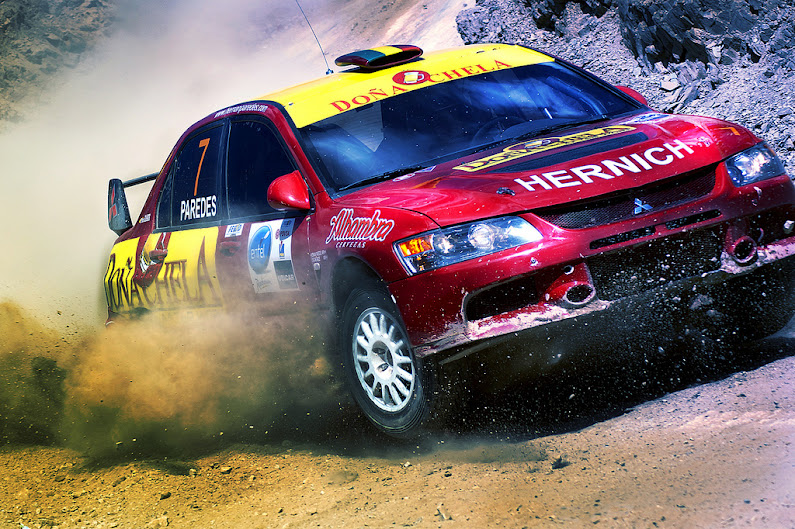Δx = vavgt               Δx = vit + ½ at2

vf = at + vi               vf2 = vi2 + 2aΔx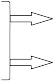The standard 4

kinematic equations.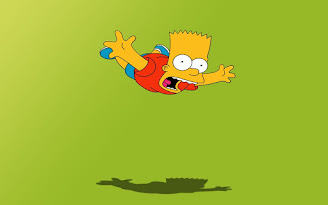A standard motion detector from the company Vernier.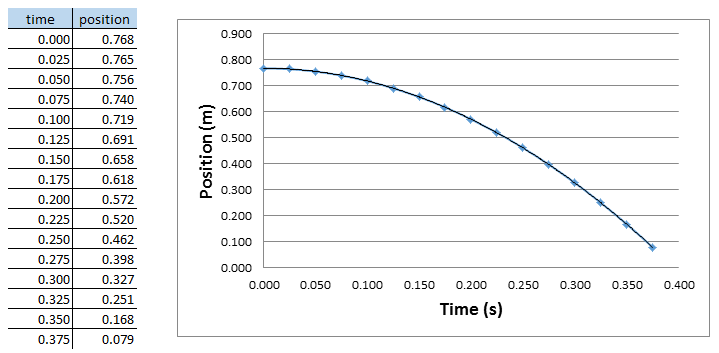After obtaining the graph, I asked the computer to generate the equation for this graph. (Both Microsoft Excel and Vernier's Logger Pro are capable of this, among other programs.) It returned this equation:

y = -4.896x2 + 0.7678

Do you remember how to interpret this?

Had the computer "realized" that position was on the vertical axis and time was on the horizontal axis, it could have returned the equation:

xf = -4.896t2 + 0.7678

Our job is to associate this equation with one of the 4 equations of motion, one of our 4 models.

y   =  0.7678              -4.896  x2

xf  =   xi         + vt  + ½ a      t

Having found the right model, we interpret the various numbers in our equation. We interpret the 0.7678 as our initial position. Apparently, the ball was 0.7678 meters above the ground (or rather above the motion detector) when it was released. The lack of a number corresponding to vit means the ball didn't have an initial velocity; it was at rest when it began to fall. And finally, -4.986 is half of the acceleration, so the ball accelerated downward at -9.792 m/s/s.

This model, written properly with direction indicated, would read:   xf = (-4.896 j)t2 + 0.7678 j

If we wanted to work out the ball's position after falling for 0.090 seconds, we could interpolate by substituting that time into our model.

xf = (-4.896 j)(0.0902) + 0.7678 j = 0.7281 j (m)

Now that we know the starting position (.7678 j), starting velocity (0) and acceleration (-9.792 j) of our ball, we can use another of our models, vf = at + vi.

vf = (-9.792 j)t

And we could use this model to find the velocity of the ball at, say, t = 0.250 seconds.

vf = (-9.792 j)(0.250) = -2.448 j (m/s)

Recognize that the velocity is negative because the ball is moving downward, along the y-axis, which is designated by j.

The acceleration is also negative, or downward, but recall that the acceleration does NOT indicate the actual direction of motion. It might be the same as the direction of motion, but it does not indicate it. The velocity indicates the direction of motion. A ball thrown upward will still have a negative acceleration, for example!

Okay, so now I'm going to show you the data and graph of a different ball I threw upward. I tried to throw it straight up, so it would rise and fall directly above the motion detector. Note that this ball is about 50% heavier than the first ball.

the distance fallen: -0.0397

the

starting

height

Δx = vit + ½ at2

becomes

xf = xi + vit + ½ at2

because

Δx = xf - xi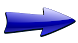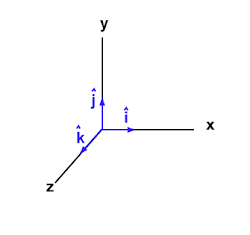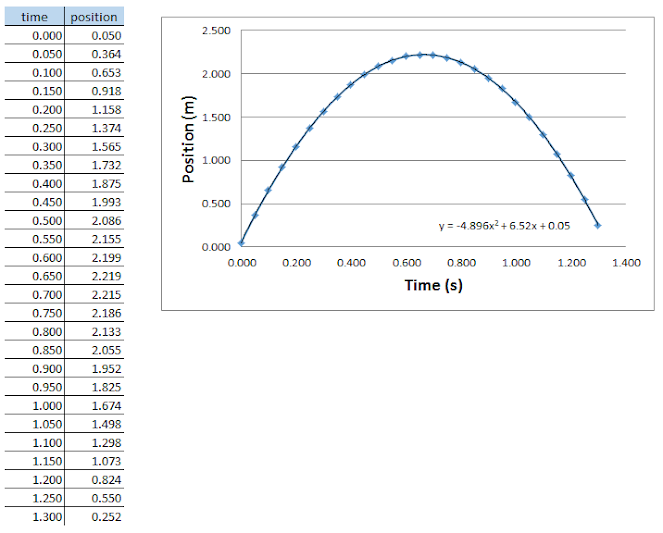I asked the computer to generate the equation for this graph; you can see it on the graph itself. Here it is:

y = -4.896x2 + 6.52x + 0.05

Let's interpret the various numbers. Let's transition from seeing this as an equation (or function) to seeing it as a model of a specific item that is moving within our world.

y   =  0.05   + 6.52 x     -4.896  x2

xf  =   xi      +  vi      t     +  ½ a   t

We see that the ball began moving upward at 6.52 m/s from an initial height of 0.05 m. As before, its acceleration is -9.792 m/s2.

Writing out our model formally, and including direction, we have:

xf = 0.05 j + (6.52 j)t + (-4.896 j)t2

(we could also write: xf = 0.05 j + (6.52 j)t - (4.896 j)t2 or change the order of the terms)

Notice that the acceleration is constant. It's always -9.792 m/s2, whether the ball is moving upward or downward. (This is good, considering that our models are only valid for constant-acceleration motion.)

Can we make sense of a constantly negative acceleration? Look at the velocity data (which I calculated using the model vf = at + vi). Notice that the velocity is always decreasing, whether the ball is moving upward or downward. The absolute value of the velocity is the speed. Notice that the speed decreases for roughly 0.650 seconds, and then it increases until the ball lands on the detector. It should be fairly obvious that the ball reaches its highest position when the velocity is momentarily zero, which happens between 0.650 and 0.700 seconds. Also, just to make it explicit, velocity is positive (+ j) when the ball is moving upward and negative (- j) when the ball is moving downward.

Recall what acceleration is actually telling us: how much the velocity changes every second. For a ball dropped, or thrown straight up, the velocity decreases by 9.792 m/s every second. In the data table to the left, notice that the velocity at 1.000 seconds (highlighted in yellow) is exactly 9.792 m/s less than one second prior. You'd also find that the velocity at 1.300 seconds is 9.792 m/s less than at 0.300 seconds, etc.

Stop and re-read the two preceding paragraphs. Really think about what they're saying.

Were you surprised that the acceleration was -9.792 m/s2 in both examples, even though the second example featured a much heavier ball?

Experimentation with many different objects reveals that they ALL accelerate at the same rate when freely falling! The mass or weight of the object doesn't influence how fast it falls.

Okay, I lied. Everything falls with the same downward acceleration only in the absence of air resistance or other interfering forces, like wind.

If you drop a feather and a hammer, the hammer falls more rapidly to the ground. The feather flutters slowly to the ground, slowed significantly by resistance from air molecules. (The hammer feels some resistance, but there is little noticeable effect.) Likewise, an apple falls to the ground more rapidly than a leaf. Based on these casual everyday observations, many people throughout history have assumed that heavier objects always fall more quickly than lighter objects. Let's take a close look at one such person.

One of the most brilliant and influential men to set foot on the Earth was born in the northeaster corner of modern Greece, in a town called Stageira (near present-day Stagira).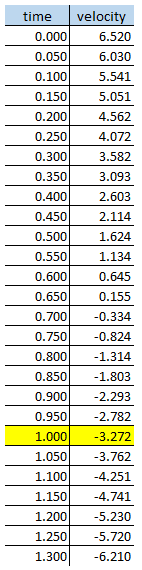The man was called Aristotle, and he was born in the year 384 BCE. At 17, he traveled to Athens to study at the famous Academy under the great philosopher Plato. He remained at the Academy, as student and later teacher, for twenty years (until the death of Plato). He then left Athens, returning in 335 BCE to found his own school, called the Lyceum. Here he did most of his writing; his interests were vast -- physics, biology, zoology, metaphysics, logic, ethics, aesthetics, poetry, theater, music, rhetoric, linguistics, politics and government.

I mention Aristotle now because he is one of the first people to attempt to systematically describe the natural world. Unlike his teacher Plato, Aristotle believed that the truth of the world was to be found here on Earth, not in some higher dimension. Aristotle spent years studying the natural world, attempting to understand how and why it operated as it does. He eventually settled upon the idea that there are "four causes" that explain existence and justify change. (Aristotle does not use the word "cause" in the same way that you and I do. To him, a "cause" is something like an explanation.) These four causes are: the material cause, or what a thing is made of; the formal cause, or the arrangement of shape of a thing; the efficient cause, or how a thing is brought into being; and the final cause, or the function or purpose of a thing. To suggest that objects in the natural world have an intrinsic purpose is known as teleology. As examples, the final cause of an eye is to see, and the final cause of an acorn is to become an oak tree. (Teleology as embraced by Aristotle was challenged by many scientists and philosophers to follow, including Immanuel Kant, who suggested that teleology is a guide to the conduct of inquiry rather than to the nature of reality.)

When it came to motion, Aristotle proposed that objects move toward their natural place. This idea is predicated on the theory of the four Classical elements, which holds that the material world (excluding "the heavens") is ultimately comprised of Earth, Water, Air and Fire. But note: We can't take this too literally, for neither did the ancients. In the words of Philip Ball, "When Plato speaks of water the element, he does not mean the same thing as the water that flows in rivers. River water is a manifestation of elementary water, but so is molten lead. Elementary water is 'that which flows'. Likewise, elementary earth is not just the stuff in the ground, but flesh, wood, metal." (from The Elements: A Very Short Introduction) To Aristotle, the elements naturally stratify, just as oil and vinegar naturally separate, with oil rising. Given bits of earth and water and air, the earth will fall downward while the air will rise. Fire rises above air. If you drop a rock into a pond, it will sink. Why? Because its natural place is below water. Thus, motion is the result of objects seeking their natural place, according to their composition.

Furthermore, according to Aristotle, earth falls downward because it is drawn to the center of the universe, which coincides with the center of the planet. (Attraction to the center of the universe was also used by Aristotle to justify his belief in the spherical nature of the Earth.)

Finally, Aristotle proposes that a falling body has a speed proportional to its weight. In his own words, "A given weight moves a given distance in a given time; a weight which is as great and more moves the same distance in a less time, the times being in inverse proportion to the weights. For instance, if one weight is twice another, it will take half as long over a given movement" (quoted from On the Heavens).

Okay, so we now have a specific claim that heavier objects always fall faster than lighter ones. (Furthermore, the falling speed is proportional to the weight.) A claim that is rational but not empirical; i.e. it is based in logic (albeit faulty) but not observation -- well, not careful observation. A claim that was accepted as fact for nearly two thousand years. A claim that is wrong.

While a few individuals realized that this claim was wrong, over the ages, they didn't understand the significance of their realization. Even if they had, the world wasn't ready to listen. It was only in the late 1600's -- nearly two thousand years after the time of Aristotle -- that a bearded man living in northern Italy (Tuscany) had the key insights, the platform, and a community (if not a Church) willing to listen.

Galileo Galilei was born in 1564 in the city of Pisa into a society undergoing profound and unsettling change. A revolution in how men viewed the world and their place within it was taking place, initiated in part by the 1543 publication of Nicolaus Copernicus's heliocentric theory. (The core of the theory was that the Earth and other planets orbited the Sun. However, it should be said that Copernicus was not the first to propose such a theory; a heliocentric theory was proposed by the Greek Aristarchus just decades after the death of Aristotle.)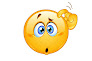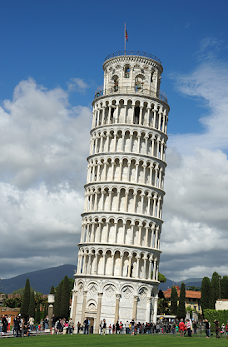A little history:

"In 1581 Galileo enrolled at the University of Pisa to study medicine but soon switched to mathematics, which he also studied privately outside the university. In 1585 he left the university without a degree and began several years of private teaching and independent research. In 1589 he was appointed professor of mathematics at the University of Pisa, and then from 1592 to 1610 at the University of Padua." (quoted from The Essential Galileo, Ed. Maurice Finocchiaro)

Galileo is considered to be one of the greatest scientists of all time. Einstein called him "the father of modern physics -- indeed of modern science altogether." Why? What did he accomplish?

The Leaning Tower of Pisa

Galileo Galilei

Yes, he disproved one of Aristotle's claims. But others had done do, as well. What sets Galileo apart from others was his approach to gaining knowledge about the world. Galileo was the first person to attempt to create mathematical models of the world, based upon data collected through experimentation. This approach invalidated the approach of Aristotle, which was based on observation, logic and intuition. Aristotle noticeably never conducted a scientific experiment. The idea of scientific experimentation as a tool for gaining knowledge about the world simply did not exist prior to the Scientific Revolution of the sixteenth and seventeenth centuries, of which Galileo was a leading figure.

Experimentation led Galileo to conclude that falling objects accelerate and the rate at which they do so is independent of their masses, at least in the absence of air resistance.

That last part, "at least in the absence of air resistance", was an Earth-shattering realization.

Galileo realized, and I quote theoretical physicist Sean Carroll of Caltech, "we can learn about the natural motion of objects by imagining we can get rid of various nuisance effects, such as friction and air resistance, and then perhaps recovering more realistic kinds of motion by putting those effects back in later." (The Big Picture) To which Carroll adds, "It is arguably the biggest idea in the history of physics."

So new was the idea that one could justify a claim (we could say support a hypothesis) about the natural world using experimental results that Galileo was reluctant to publicly use such evidence in defense of his claims. In his final book, Discourses and Mathematical Demonstrations Relating to Two New Sciences, published in 1638, Galileo presents his conclusions on the nature of falling bodies, yet he supports these conclusions by means of a logical argument.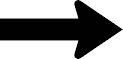What follows is a passage from Galileo's Two New Sciences, laid out as a conversation among three people.

Salviati:

"... I greatly doubt that Aristotle ever tested by experiment whether it be true that two stones, one weighing ten times as much as the other, if allowed to fall at the same instant from a height of say, one hundred cubits, would so differ in speed that when the heavier had reached the ground, the other would not have fallen more than ten cubits.

Simplicio:

"His language would seem to indicate that he had tried the experiment, because he says, "we see the heavier"; now the word see shows that he had made the experiment."

Sagredo:

"But I, Simplicio, who have made the test can assure you that a cannon ball weighing one or two hundred pounds, or even more, will not reach the ground by as much as a span ahead of a musket ball weighing only half a pound, provided both are dropped from a height of two hundred cubits."

Salviati:

"But, even without further experiment, it is possible to prove clearly, by means of a short and conclusive argument, that a heavier body does not move more rapidly than a lighter one, provided both bodies are of the same material -- in short, such as those mentioned by Aristotle."

Salviati then goes on to argue logically that a heavier body cannot fall faster than a lighter body.

Okay, so let's summarize.

In the absence of air resistance, all objects accelerate downward at the same rate. The size, shape, weight or composition of the object does not affect how quickly it falls. And from the data I collected earlier, from the ball thrown upward above the motion detector, that unique downward acceleration is -9.792 m/s2. Well, it's -9.792 m/s2 in Austin, Texas.And it's -9.804 m/s2 in New York City.In the absence of air resistance, anything dropped from the 102nd floor observatory of the Empire State Building (pictured at left) would hit the ground below (assuming it didn't hit the side of the building) with a speed of 86.4 m/s (or 193 mph)!

Air resistance reduces this speed considerably.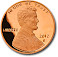And it's -9.813 m/s2 in Paris.And it's -9.796 m/s2 in Sydney.The slight variation in free-fall acceleration, or local acceleration of gravity, as it's called, will be explored later, but it has to do with the slight difference in distance between each of these cities and the center of the Earth.

Nonetheless, we can comfortably say that objects around the Earth, in the absence of air resistance, fall downward at -9.8 m/s2. And even with air resistance, many objects fall at about that same rate.

When developing your intuition for how objects fall, however, it is helpful to approximate this acceleration as -10 m/s2. This is widely done, and we'll use this approximation below.

Take a look at the data collected for an object on Earth (it doesn't matter what it is) that is falling for 10 seconds. I've fabricated this data, assuming an acceleration of -10 m/s/s. Yes, the data excludes the effects of air resistance. Since the time of Galileo, physicists have regularly ignored "various nuisance effects" in an effort to simplify model-building and illuminate otherwise hidden patterns.

What do you notice?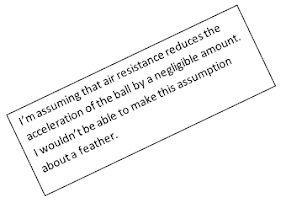the local acceleration  of gravity in your city.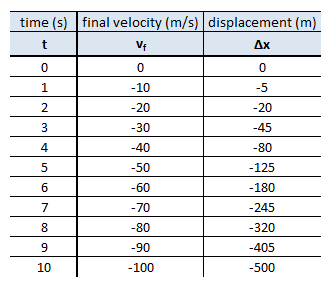If I told you that a falling object, dropped from rest, was moving downward at -160 m/s, could you tell me for how many seconds it had been falling?  What about if it had a velocity of -25 m/s?

The model illustrated here is simply vf = at + vi, where vi happens to be zero (indicating the object was dropped from rest). Given that the model is representing a falling object, and experimental data indicates that such objects (on Earth) accelerate at about -10 m/s2, we could update the model to reflect this information.

Thus, vf = (-10 j)t. (Recall that j is simply an indication of direction.)

Notice how the displacement is growing, by larger and larger amounts each second. Realize this is a running total. In the first second, the object falls 5 m, in the next second, it falls an additional 15 m, for a total of 20 m. This happens when things accelerate.

The model that best illustrates this relationship between displacement and time is none other than

Δx = vit + ½ at2, where vi is zero and, as before, a = -10 m/s2. It simplifies here to Δx = (-5 j)t2.

The illustration at left should help you to visualize the falling. It represents 4 seconds. As you can see, I've labeled the additional distance traveled each second, not the total. If you calculate the totals, they'll match the data in the table above. (Notice a pattern?)

Now take a look at the data for a ball thrown upward at 40 m/s. Try to make sense of it.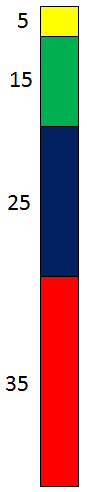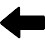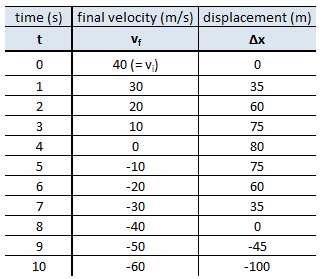Try to answer the following questions:

What is the ball's speed (or velocity) at its peak (highest point)?

If the ball had been thrown upward at 50 m/s, how many seconds would it have taken to reach its peak?

Using the data table, at what time does the ball return to its starting height? (Notice the symmetry?)

If the ball had been thrown upward at 60 m/s, how many seconds would have elapsed before it returned to its starting height?

How does the ball's initial speed compare to its speed when it returns to its starting height?

Realize that the only way we could have collected this data was if the person throwing the ball did so over a hole or cliff. In those last 2 seconds, the ball falls 100 m below its starting height.

Here are our two models:

vf = (-10 j)t + 40 j

Δx = (40 j)t + (-5 j)t2

Finally, let's take a look at the data for a ball thrown downward at 20 m/s.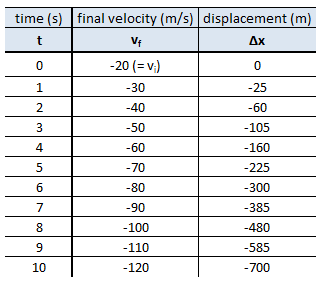You might think that a ball thrown downward will accelerate downward at a rate different than -10 m/s2. It doesn't, after it's left your hand. Once "gravity takes over", acceleration will always be -10 m/s2. Whatever the velocity is, it will decrease by 10 m/s2 for every second of falling, as you see in the data above.

I want to reference another of our models, to show you that they're all valid all of the time, as long as acceleration is constant (although at times certain models are not useful).

Δx = vavgt

To find the displacement over the first 2 seconds, we can find the average velocity over that time and multiply it by the number of seconds that passed.

The displacement over the first 5 seconds is:

Once more. Let's find the displacement that occurs between t = 5 and t = 8 seconds. (We'll use t = 3 in our model, because it represents the time interval.)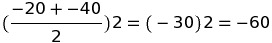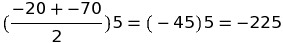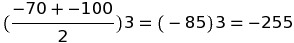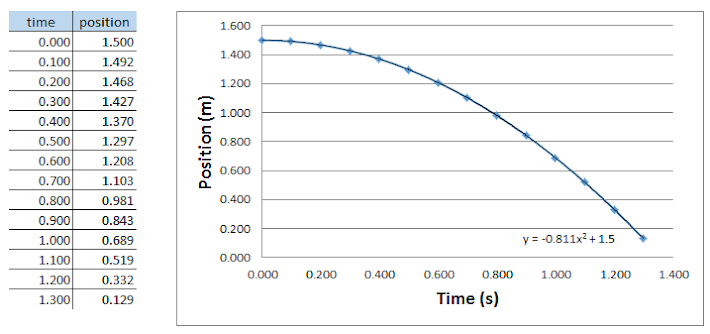can you work out the local acceleration of gravity o n the moon?

This data tracks a falling object on the Moon. (Units are seconds and meters.)

Using the equation printed on the graph, determine the acceleration of gravity on the Moon.

Was the object dropped from rest or thrown?

From what height was it released?

Astronaut John Young jumps for the camera during the Apollo 16 mission.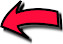In the absence of air resistance, objects will fall at the same rate.

What better place to test this hypothesis than the Moon, which lacks an atmosphere.

Watch astronaut David Scott, here standing on the Moon (Apollo 15, 1971), drop a heavy hammer and light feather. Do they land at the same time?practice  problems

problems -

interpreting trend-

lines and applying

models

problems -

based on initial velocity

(mostly mental math)